# Basic Operations in Octave

GNU Octave is a high-level programming language, primarily intended for numerical computations. It can also be used to implement various machine learning algorithms with ease. Octave is open-source i.e. it is free to use, whereas MATLAB is not thus MATLAB requires a licence to operate.

Below are the various basic functionalities of Octave :

1. Arithmetic Operations : Octave can be used to perform basic mathematical operations like addition, subtraction, multiplication, power operation etc.

 `     `  `% addition operation ` `23 + 65 + 8 ` ` `  `% subtraction operation ` `32 - 74 ` ` `  `% power operation ` `6 ^ 2 ` ` `  `% multiplication operation ` `45 * 7 ` ` `  `% division operation ` `5 / 6 `

Output :

```ans = 96
ans = -42
ans = 36
ans = 315
ans = 0.83333
```

2. Logical Operations : Octave can be used to perform logical operations like AND, OR, NOT etc.

 `  `  `% logical AND      ` `1 && 0 ` ` `  `% logical OR ` `1 || 0 ` ` `  `% logical NOT ` `~1 `

Output :

```ans = 0
ans = 1
ans = 0
```

3. Relational Operations : Octave can be used to perform relational operations like greater than, less than etc.

 `  `  `% equal to ` `1 == 1 ` ` `  `% not equal to ` `0 ~= 0 ` ` `  `% greater than     ` `1 > 0 ` ` `  `% less than ` `1 < 0 ` ` `  `% greater than equal to   ` `1 >= 2 ` ` `  `% less than equal to ` `0 <= 0 `

Output :

```ans = 1
ans = 0
ans = 1
ans = 0
ans = 0
ans = 1
```

4. Changing the default Octave prompt symbol : The default Octave prompt symbol is “>>”. We can change the default Octave prompt symbol using the below commands :

 `    `  `PS1(``'<< '``); ` `PS1(``'@ '``); ` `PS1(``'# '``); `

Output :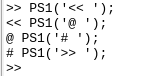5. Variables : Like other programming languages, Octave also has variables to temporarily store data.

 `     `  `% variable declaration and initialization ` `var = 2 ` ` `  `% if we want to create the variable and don't want to print it ` `% then put a semicolon at the end of that command ` `var = 3; ``% this time the variable will not be printed ` ` `  `% variable of datatype char  ` `ch = ``'c'` ` `  `% storing the result of an operation in a variable ` `res = (1 != 1) ` ` `  `% storing the value of pi in a varible ` `var = pi ` ` `  `% printing a variable with disp() function ` `disp(var); ` ` `  `% using sprintf() function to print a string ` `disp(sprintf(``'3 decimal values : %0.3f'``, var)) ` ` `  `% using format long to resize ` `format long ` `var ` ` `  `% using format short to resize ` `format short ` `var `

Output :

```var =  2
ch = c
res = 0
var =  3.1416
3.1416
3 decimal values : 3.142
var =  3.141592653589793
var =  3.1416
```

6. Matrices and Vectors : Now let’s learn how to deal with matrices and vectors in Octave. We can create matrix as shown below.

 `   `  `% creating matrix in row major  ` `matrix = [1 2 3; 4 5 6; 7 8 9] `

Output :

```matrix =

1   2   3
4   5   6
7   8   9
```

We can also make a vector, a vector is a matrix with n rows and 1 column(column vector) or 1 rows with n columns(row vector). here in example 2 and 3 the middle value 5 and 0.5 shows that we want to make a vector matrix from range 1 to 20 with the jump of 5 and from range 0 to 5 with a jump of 0.5 respectively.

 `    `  `% creating row vector ` `r_v = [1, 2, 3] ` ` `  `% creating column vector ` `c_v = [1; 2; 3] `

Output :

```r_v =

1   2   3

c_v =

1
2
3
```

Here are some utility shortcuts to create matrices and vectors :

 `% creating vector using ":" ` `% the extreme end values denote the range ` `% and the middle value denotes the step ` `v1 = 1 : 5 : 20 ` `v2 = 1 : 0.5 : 5 ` ` `  `% without the step parameter ` `v3 = 1 : 10 ` ` `  `% generate matrix of size 4x4 with all element as 1 ` `ones_matrix = ones(4, 4) ` ` `  `% generate matrix of size 4x4 with all element as 10 ` `M = 10 * ones(4, 4) ` ` `  `% generate row vector of size 5 with all elements 0 ` `zeroes_vector = zeros(1, 5) ` ` `  `% generate row vector of some random numbers between 0 and 1 ` `random_vector = rand(1, 5) ` ` `  `% generate matrix of some random numbers between 0 and 1 ` `random_matrix = rand(3, 4) ` ` `  `% generate matrix with Gaussian distribution ` `% where mean = 0 and variance and standard deviation = 1 ` `gauss_matrix = randn(5, 5) ` ` `  `% generate identity matrix with size 5x5 ` `identity_matrix = eye(5) `

Output :

```v1 =

1    6   11   16

v2 =

1.0000    1.5000    2.0000    2.5000    3.0000    3.5000    4.0000    4.5000    5.0000

v3 =

1    2    3    4    5    6    7    8    9   10

ones_matrix =

1   1   1   1
1   1   1   1
1   1   1   1
1   1   1   1

M =

10   10   10   10
10   10   10   10
10   10   10   10
10   10   10   10

zeroes_vector =

0   0   0   0   0

random_vector =

0.79085   0.35395   0.92267   0.60234   0.75549

random_matrix =

0.64434   0.67677   0.54105   0.83149
0.70150   0.16149   0.38742   0.90442
0.60075   0.82273   0.37113   0.91496

gauss_matrix =

0.705921   1.336101  -0.097530   0.498245   1.125928
-0.550047  -1.868716  -0.977788   0.319715  -0.603599
-0.018352  -2.133200   0.462272   0.169707   1.733255
0.623343   0.338734   0.618943   1.110172   1.731495
-1.741052  -0.463446   0.556348   1.633956  -1.424136

identity_matrix =

Diagonal Matrix

1   0   0   0   0
0   1   0   0   0
0   0   1   0   0
0   0   0   1   0
0   0   0   0   1
```

7. Histograms : We can draw the histograms `hist()` function. We can also change the bucket size or bins of the histogram.

 `  `  `% generate a vector with 1000 elements ` `elements_1000 = 1 + sqrt(25)*(randn(1, 1000)); ` ` `  `hist(elements_1000 ) `

Output :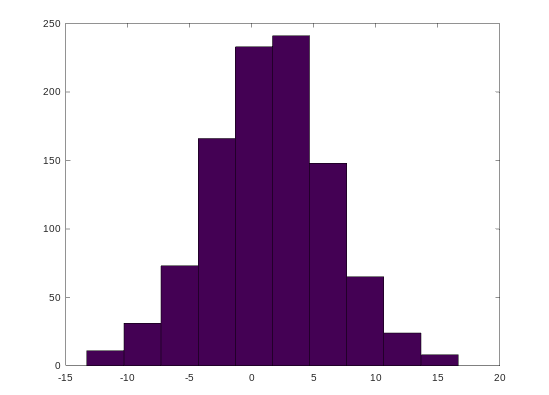`  `  `% generate a vector with 1000 elements ` `elements_1000 = 1 + sqrt(25)*(randn(1, 1000)); ` ` `  `% histogram with 30 bins ` `hist(elements_1000, 30) `

Output :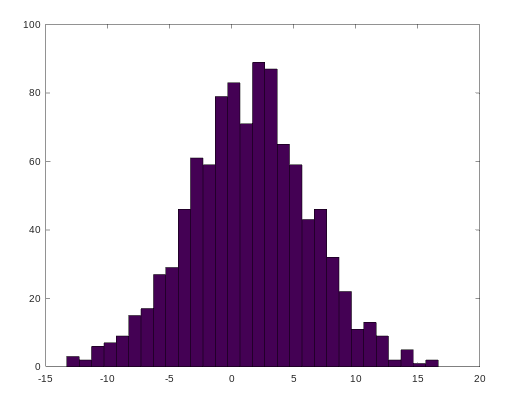8. Help : We can use the `help` command to see the documentation for any function.

 `  `  `help eye ` `help sqrt ` `help hist `

Output :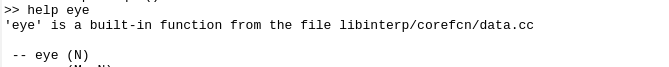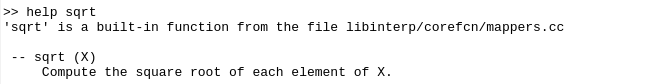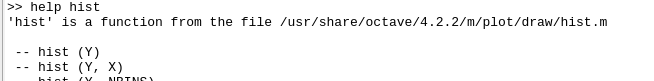My Personal Notes arrow_drop_upCheck out this Author's contributed articles.

If you like GeeksforGeeks and would like to contribute, you can also write an article using contribute.geeksforgeeks.org or mail your article to contribute@geeksforgeeks.org. See your article appearing on the GeeksforGeeks main page and help other Geeks.

Please Improve this article if you find anything incorrect by clicking on the "Improve Article" button below.

Article Tags :

Be the First to upvote.

Please write to us at contribute@geeksforgeeks.org to report any issue with the above content.